# alternating current 2

When an alternator produces AC voltage, the voltage switches polarity over time, but does so in a very particular manner. When graphed over time, the “wave” traced by this voltage of alternating polarity from an alternator takes on a distinct shape, known as a sine wave: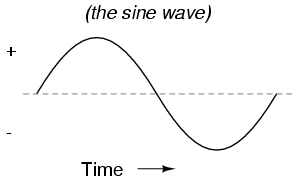Graph of AC voltage over time (the sine wave).
In the voltage plot from an electromechanical alternator, the change from one polarity to the other is a smooth one, the voltage level changing most rapidly at the zero (“crossover”) point and most slowly at its peak. If we were to graph the trigonometric function of “sine” over a horizontal range of 0 to 360 degrees, we would find the exact same pattern as in Table
Trigonometric “sine” function.

Angle (o) sin(angle) wave Angle (o) sin(angle) wave
0 0.0000 zero 180 0.0000 zero
15 0.2588 + 195 -0.2588
30 0.5000 + 210 -0.5000
45 0.7071 + 225 -0.7071
60 0.8660 + 240 -0.8660
75 0.9659 + 255 -0.9659
90 1.0000 +peak 270 -1.0000 -peak
105 0.9659 + 285 -0.9659
120 0.8660 + 300 -0.8660
135 0.7071 + 315 -0.7071
150 0.5000 + 330 -0.5000
165 0.2588 + 345 -0.2588
180 0.0000 zero 360 0.0000 zero

The reason why an electromechanical alternator outputs sine-wave AC is due to the physics of its operation. The voltage produced by the stationary coils by the motion of the rotating magnet is proportional to the rate at which the magnetic flux is changing perpendicular to the coils (Faraday’s Law of Electromagnetic Induction). That rate is greatest when the magnet poles are closest to the coils, and least when the magnet poles are furthest away from the coils. Mathematically, the rate of magnetic flux change due to a rotating magnet follows that of a sine function, so the voltage produced by the coils follows that same function.
If we were to follow the changing voltage produced by a coil in an alternator from any point on the sine wave graph to that point when the wave shape begins to repeat itself, we would have marked exactly one cycle of that wave. This is most easily shown by spanning the distance between identical peaks, but may be measured between any corresponding points on the graph. The degree marks on the horizontal axis of the graph represent the domain of the trigonometric sine function, and also the angular position of our simple two-pole alternator shaft as it rotates: Figure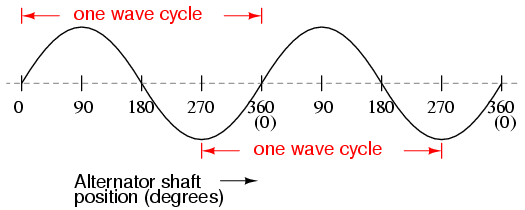Alternator voltage as function of shaft position (time).
Since the horizontal axis of this graph can mark the passage of time as well as shaft position in degrees, the dimension marked for one cycle is often measured in a unit of time, most often seconds or fractions of a second. When expressed as a measurement, this is often called the period of a wave. The period of a wave in degrees is always 360, but the amount of time one period occupies depends on the rate voltage oscillates back and forth.
A more popular measure for describing the alternating rate of an AC voltage or current wave than period is the rate of that back-and-forth oscillation. This is called frequency. The modern unit for frequency is the Hertz (abbreviated Hz), which represents the number of wave cycles completed during one second of time. In the United States of America, the standard power-line frequency is 60 Hz, meaning that the AC voltage oscillates at a rate of 60 complete back-and-forth cycles every second. In Europe, where the power system frequency is 50 Hz, the AC voltage only completes 50 cycles every second. A radio station transmitter broadcasting at a frequency of 100 MHz generates an AC voltage oscillating at a rate of 100 million cycles every second.
Prior to the canonization of the Hertz unit, frequency was simply expressed as “cycles per second.” Older meters and electronic equipment often bore frequency units of “CPS” (Cycles Per Second) instead of Hz. Many people believe the change from self-explanatory units like CPS to Hertz constitutes a step backward in clarity. A similar change occurred when the unit of “Celsius” replaced that of “Centigrade” for metric temperature measurement. The name Centigrade was based on a 100-count (“Centi-”) scale (“-grade”) representing the melting and boiling points of H2O, respectively. The name Celsius, on the other hand, gives no hint as to the unit’sorigin or meaning.
Period and frequency are mathematical reciprocals of one another. That is to say, if a wave has a period of 10 seconds, its frequency will be 0.1 Hz, or 1/10 of a cycle per second:An instrument called an oscilloscope, Figure , is used to display a changing voltage over time on a graphical screen. You may be familiar with the appearance of an ECG or EKG (electrocardiograph) machine, used by physicians to graph the oscillations of a patient’s heart over time. The ECG is a special-purpose oscilloscope expressly designed for medical use. General-purpose oscilloscopes have the ability to display voltage from virtually any voltage source, plotted as a graph with time as the independent variable. The relationship between period and frequency is very useful to know when displaying an AC voltage or current waveform on an oscilloscope screen. By measuring the period of the wave on the horizontal axis of the oscilloscope screen and reciprocating that time value (in seconds), you can determine the frequency in Hertz.Time period of sinewave is shown on oscilloscope.
Voltage and current are by no means the only physical variables subject to variation over time. Much more common to our everyday experience is sound, which is nothing more than the alternating compression and decompression (pressure waves) of air molecules, interpreted by our ears as a physical sensation. Because alternating current is a wave phenomenon, it shares many of the properties of other wave phenomena, like sound. For this reason, sound (especially structured music) provides an excellent analogy for relating AC concepts.
In musical terms, frequency is equivalent to pitch. Low-pitch notes such as those produced by a tuba or bassoon consist of air molecule vibrations that are relatively slow (low frequency). High-pitch notes such as those produced by a flute or whistle consist of the same type of vibrations in the air, only vibrating at a much faster rate (higher frequency). Figure is a table showing the actual frequencies for a range of common musical notes.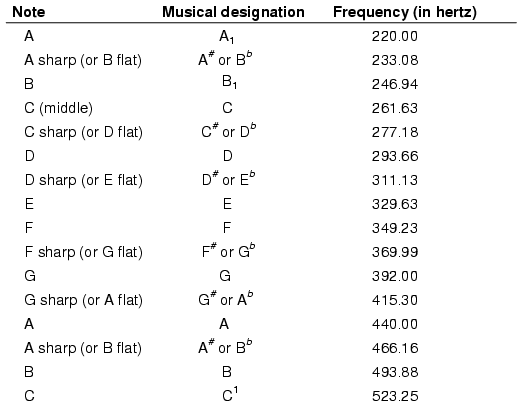The frequency in Hertz (Hz) is shown for various musical notes.
Astute observers will notice that all notes on the table bearing the same letter designation are related by a frequency ratio of 2:1. For example, the first frequency shown (designated with the letter “A”) is 220 Hz. The next highest “A” note has a frequency of 440 Hz — exactly twice as many sound wave cycles per second. The same 2:1 ratio holds true for the first A sharp (233.08 Hz) and the next A sharp (466.16 Hz), and for all note pairs found in the table.
Audibly, two notes whose frequencies are exactly double each other sound remarkably similar. This similarity in sound is musically recognized, the shortest span on a musical scale separating such note pairs being called an octave. Following this rule, the next highest “A” note (one octave above 440 Hz) will be 880 Hz, the next lowest “A” (one octave below 220 Hz) will be 110 Hz. A view of a piano keyboard helps to put this scale into perspective: Figure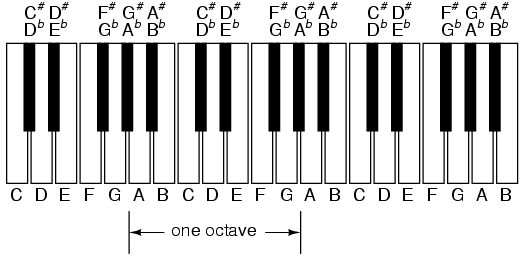An octave is shown on a musical keyboard.
As you can see, one octave is equal to seven white keys’ worth of distance on a piano keyboard. The familiar musical mnemonic (doe-ray-mee-fah-so-lah-tee) — yes, the same pattern immortalized in the whimsical Rodgers and Hammerstein song sung in The Sound of Music — covers one octave from C to C.
While electromechanical alternators and many other physical phenomena naturally produce sine waves, this is not the only kind of alternating wave in existence. Other “waveforms” of AC are commonly produced within electronic circuitry. Hre are but a few sample waveforms and their common designations in figureSome common waveshapes (waveforms).
These waveforms are by no means the only kinds of waveforms in existence. They’re simply a few that are common enough to have been given distinct names. Even in circuits that are supposed to manifest “pure” sine, square, triangle, or sawtooth voltage/current waveforms, the real-life result is often a distorted version of the intended waveshape. Some waveforms are so complex that they defy classification as a particular “type” (including waveforms associated with many kinds of musical instruments). Generally speaking, any waveshape bearing close resemblance to a perfect sine wave is termed sinusoidal, anything different being labeled as non-sinusoidal. Being that the waveform of an AC voltage or current is crucial to its impact in a circuit, we need to be aware of the fact that AC waves come in a variety of shapes.

• REVIEW:
• AC produced by an electromechanical alternator follows the graphical shape of a sine wave.
• One cycle of a wave is one complete evolution of its shape until the point that it is ready to repeat itself.
• The period of a wave is the amount of time it takes to complete one cycle.
• Frequency is the number of complete cycles that a wave completes in a given amount of time. Usually measured in Hertz
(Hz), 1 Hz being equal to one complete wave cycle per second.
• Frequency = 1/(period in seconds)

## Measurements of AC magnitude

So far we know that AC voltage alternates in polarity and AC current alternates in direction. We also know that AC can alternate in a variety of different ways, and by tracing the alternation over time we can plot it as a “waveform.” We can measure the rate of alternation by measuring the time it takes for a wave to evolve before it repeats itself (the “period”), and express this as cycles per unit time, or “frequency.” In music, frequency is the same as pitch, which is the essential property distinguishing one note from another.
However, we encounter a measurement problem if we try to express how large or small an AC quantity is. With DC, where quantities of voltage and current are generally stable, we have little trouble expressing how much voltage or current we have in any part of a circuit. But how do you grant a single measurement of magnitude to something that is constantly changing?
One way to express the intensity, or magnitude (also called the amplitude), of an AC quantity is to measure its peak height on a waveform graph. This is known as the peak or crest value of an AC waveform: Figure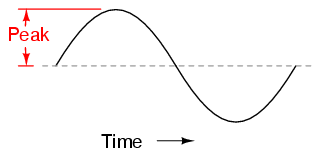Peak voltage of a waveform.
Another way is to measure the total height between opposite peaks. This is known as the peak-to-peak (P-P) value of an AC waveform:Peak-to-peak voltage of a waveform.
Unfortunately, either one of these expressions of waveform amplitude can be misleading when comparing two different types of waves. For example, a square wave peaking at 10 volts is obviously a greater amount of voltage for a greater amount of time than a triangle wave peaking at 10 volts. The effects of these two AC voltages powering a load would be quite different: Figure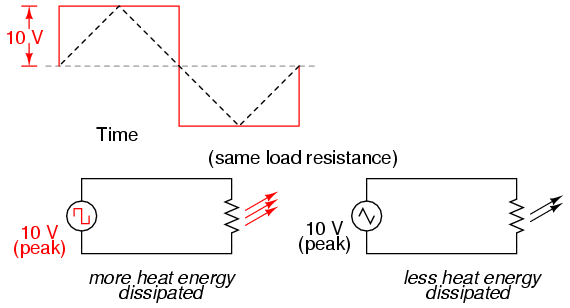A square wave produces a greater heating effect than the same peak voltage triangle wave.
One way of expressing the amplitude of different waveshapes in a more equivalent fashion is to mathematically average the values of all the points on a waveform’s graph to a single, aggregate number. This amplitude measure is known simply as the average value of the waveform. If we average all the points on the waveform algebraically (that is, to consider their sign, either positive or negative), the average value for most waveforms is technically zero, because all the positive points cancel out all the negative points over a full cycle: FigureThe average value of a sinewave is zero.
This, of course, will be true for any waveform having equal-area portions above and below the “zero” line of a plot.
However, as a practical measure of a waveform’s aggregate value, “average” is usually defined as the mathematical
mean of all the points’ absolute values over a cycle. In other words, we calculate the practical average value of the waveform
by considering all points on the wave as positive quantities, as if the waveform looked like this: FigureWaveform seen by AC “average responding” meter.
Polarity-insensitive mechanical meter movements (meters designed to respond equally to the positive and negative half-cycles of an
alternating voltage or current) register in proportion to the waveform’s (practical) average value, because the inertia of the pointer
against the tension of the spring naturally averages the force produced by the varying voltage/current values over time. Conversely,
polarity-sensitive meter movements vibrate uselessly if exposed to AC voltage or current, their needles oscillating rapidly about the
zero mark, indicating the true (algebraic) average value of zero for a symmetrical waveform. When the “average” value of
a waveform is referenced in this text, it will be assumed that the “practical” definition of average is intended unless
otherwise specified.
Another method of deriving an aggregate value for waveform amplitude is based on the waveform’s ability to do useful work when applied
to a load resistance. Unfortunately, an AC measurement based on work performed by a waveform is not the same as that waveform’s
“average” value, because the power dissipated by a given load (work performed per unit time) is not directly
proportional to the magnitude of either the voltage or current impressed upon it. Rather, power is proportional to the square
of the voltage or current applied to a resistance (P = E2/R, and P = I2R). Although the mathematics of such an
amplitude measurement might not be straightforward, the utility of it is.
Consider a bandsaw and a jigsaw, two pieces of modern woodworking equipment. Both types of saws cut with a thin, toothed,
motor-powered metal blade to cut wood. But while the bandsaw uses a continuous motion of the blade to cut, the jigsaw uses a
back-and-forth motion. The comparison of alternating current (AC) to direct current (DC) may be likened to the comparison of these
two saw types: Figure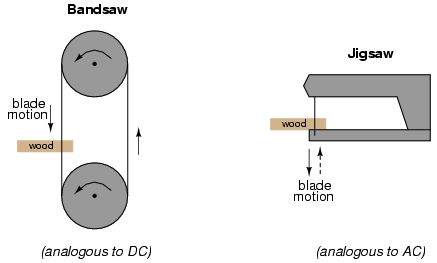Bandsaw-jigsaw analogy of DC vs AC.
The problem of trying to describe the changing quantities of AC voltage or current in a single, aggregate measurement is also present
in this saw analogy: how might we express the speed of a jigsaw blade? A bandsaw blade moves with a constant speed, similar to the
way DC voltage pushes or DC current moves with a constant magnitude. A jigsaw blade, on the other hand, moves back and forth, its
blade speed constantly changing. What is more, the back-and-forth motion of any two jigsaws may not be of the same type, depending on
the mechanical design of the saws. One jigsaw might move its blade with a sine-wave motion, while another with a triangle-wave
motion. To rate a jigsaw based on its peak blade speed would be quite misleading when comparing one jigsaw to another (or a
jigsaw with a bandsaw!). Despite the fact that these different saws move their blades in different manners, they re equal in one
respect: they all cut wood, and a quantitative comparison of this common function can serve as a common basis for which to rate blade
speed.
Picture a jigsaw and bandsaw side-by-side, equipped with identical blades (same tooth pitch, angle, etc.), equally capable of cutting
the same thickness of the same type of wood at the same rate. We might say that the two saws were equivalent or equal in their
cutting capacity. Might this comparison be used to assign a “bandsaw equivalent” blade speed to the jigsaw’s
back-and-forth blade motion; to relate the wood-cutting effectiveness of one to the other? This is the general idea used to assign a
“DC equivalent” measurement to any AC voltage or current: whatever magnitude of DC voltage or current would produce the
same amount of heat energy dissipation through an equal resistance:FigureAn RMS voltage produces the same heating effect as a the same DC voltage
In the two circuits above, we have the same amount of load resistance (2 Ω) dissipating the same amount of power in the form of
heat (50 watts), one powered by AC and the other by DC. Because the AC voltage source pictured above is equivalent (in terms of power
delivered to a load) to a 10 volt DC battery, we would call this a “10 volt” AC source. More specifically, we would
denote its voltage value as being 10 volts RMS. The qualifier “RMS” stands for Root Mean Square, the
algorithm used to obtain the DC equivalent value from points on a graph (essentially, the procedure consists of squaring all the
positive and negative points on a waveform graph, averaging those squared values, then taking the square root of that average to
obtain the final answer). Sometimes the alternative terms equivalent or DC equivalent are used instead of
“RMS,” but the quantity and principle are both the same.
RMS amplitude measurement is the best way to relate AC quantities to DC quantities, or other AC quantities of differing waveform
shapes, when dealing with measurements of electric power. For other considerations, peak or peak-to-peak measurements may be the best
to employ. For instance, when determining the proper size of wire (ampacity) to conduct electric power from a source to a load, RMS
current measurement is the best to use, because the principal concern with current is overheating of the wire, which is a function of
power dissipation caused by current through the resistance of the wire. However, when rating insulators for service in high-voltage
AC applications, peak voltage measurements are the most appropriate, because the principal concern here is insulator
“flashover” caused by brief spikes of voltage, irrespective of time.
Peak and peak-to-peak measurements are best performed with an oscilloscope, which can capture the crests of the waveform with a high
degree of accuracy due to the fast action of the cathode-ray-tube in response to changes in voltage. For RMS measurements, analog
meter movements (D’Arsonval, Weston, iron vane, electrodynamometer) will work so long as they have been calibrated in RMS figures.
Because the mechanical inertia and dampening effects of an electromechanical meter movement makes the deflection of the needle
naturally proportional to the average value of the AC, not the true RMS value, analog meters must be specifically calibrated
(or mis-calibrated, depending on how you look at it) to indicate voltage or current in RMS units. The accuracy of this calibration
depends on an assumed waveshape, usually a sine wave.
Electronic meters specifically designed for RMS measurement are best for the task. Some instrument manufacturers have designed
ingenious methods for determining the RMS value of any waveform. One such manufacturer produces “True-RMS” meters with a
tiny resistive heatingelement powered by a voltage proportional to that being measured. The heating effect of that resistance
element is measured thermally to give a true RMS value with no mathematical calculations whatsoever, just the laws of physics in
action in fulfillment of the definition of RMS. The accuracy of this type of RMS measurement is independent of waveshape.
For “pure” waveforms, simple conversion coefficients exist for equating Peak, Peak-to-Peak, Average (practical, not
algebraic), and RMS measurements to one another: Figure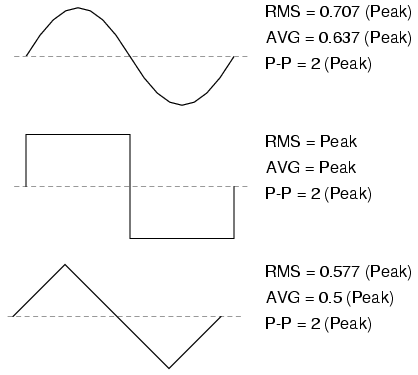Conversion factors for common waveforms.
In addition to RMS, average, peak (crest), and peak-to-peak measures of an AC waveform, there are ratios expressing the
proportionality between some of these fundamental measurements. The crest factor of an AC waveform, for instance, is the ratio
of its peak (crest) value divided by its RMS value. The form factor of an AC waveform is the ratio of its RMS value divided by
its average value. Square-shaped waveforms always have crest and form factors equal to 1, since the peak is the same as the RMS and
average values. Sinusoidal waveforms have an RMS value of 0.707 (the reciprocal of the square root of 2) and a form factor of 1.11
(0.707/0.636). Triangle- and sawtooth-shaped waveforms have RMS values of 0.577 (the reciprocal of square root of 3) and form factors
of 1.15 (0.577/0.5).
Bear in mind that the conversion constants shown here for peak, RMS, and average amplitudes of sine waves, square waves, and triangle
waves hold true only for pure forms of these waveshapes. The RMS and average values of distorted waveshapes are not related by
the same ratios: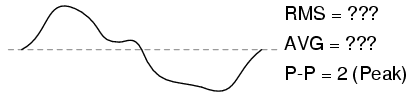Arbitrary waveforms have no simple conversions.
This is a very important concept to understand when using an analog meter movement to measure AC voltage or current. An analog
movement, calibrated to indicate sine-wave RMS amplitude, will only be accurate when measuring pure sine waves. If the waveform of
the voltage or current being measured is anything but a pure sine wave, the indication given by the meter will not be the true RMS
value of the waveform, because the degree of needle deflection in an analog meter movement is proportional to the average value
of the waveform, not the RMS. RMS meter calibration is obtained by “skewing” the span of the meter so that it displays a
small multiple of the average value, which will be equal to be the RMS value for a particular waveshape and a particular waveshape
only
.
Since the sine-wave shape is most common in electrical measurements, it is the waveshape assumed for analog meter calibration, and the
small multiple used in the calibration of the meter is 1.1107 (the form factor: 0.707/0.636: the ratio of RMS divided by average for a
sinusoidal waveform). Any waveshape other than a pure sine wave will have a different ratio of RMS and average values, and thus a
meter calibrated for sine-wave voltage or current will not indicate true RMS when reading a non-sinusoidal wave. Bear in mind that
this limitation applies only to simple, analog AC meters not employing “True-RMS” technology.

• REVIEW:
• The amplitude of an AC waveform is its height as depicted on a graph over time. An amplitude measurement can take the form
of peak, peak-to-peak, average, or RMS quantity.
• Peak amplitude is the height of an AC waveform as measured from the zero mark to the highest positive or lowest negative
point on a graph. Also known as the crest amplitude of a wave.
• Peak-to-peak amplitude is the total height of an AC waveform as measured from maximum positive to maximum negative peaks on
a graph. Often abbreviated as “P-P”.
• Average amplitude is the mathematical “mean” of all a wavform’s points over the period of one cycle.
Technically, the average amplitude of any waveform with equal-area portions above and below the “zero” line on a graph is
zero. However, as a practical measure of amplitude, a waveform’s average value is often calculated as the mathematical mean of all
the points’ absolute values (taking all the negative values and considering them as positive). For a sine wave, the average
value so calculated is approximately 0.637 of its peak value.
• “RMS” stands for Root Mean Square, and is a way of expressing an AC quantity of voltage or current in terms
functionally equivalent to DC. For example, 10 volts AC RMS is the amount of voltage that would produce the same amount of heat
dissipation across a resistor of given value as a 10 volt DC power supply. Also known as the “equivalent” or “DC
equivalent” value of an AC voltage or current. For a sine wave, the RMS value is approximately 0.707 of its peak value.
• The crest factor of an AC waveform is the ratio of its peak (crest) to its RMS value.
• The form factor of an AC waveform is the ratio of its RMS value to its average value.
• Analog, electromechanical meter movements respond proportionally to the average value of an AC voltage or current. When
RMS indication is desired, the meter’s calibration must be “skewed” accordingly. This means that the accuracy of an
electromechanical meter’s RMS indication is dependent on the purity of the waveform: whether it is the exact same waveshape as the
waveform used in calibrating.

Related

COMMENT songs